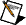# TMAX Setting Guidelines

## Multisim Help

Edition Date: February 2017
Part Number: 375482B-01
View Product InfoMultisim 14.0 and 14.0.1 HelpMultisim 14.1 HelpMultisim 14.2 Help

To achieve optimal results when running a transient simulation, the maximum timestep (TMAX) parameter must be set appropriately.

TMAX specifies the upper limit on the simulator step size (the distance between any two adjacent time points.) If the TMAX value is too large, a simulation may still converge. However, the results could differ substantially from the expected analytic results (for example, the oscillation frequency may not be correct.) To minimize errors and improve simulation accuracy, it may be necessary to manually set a custom value for TMAX.

By default, Multisim is configured to automatically determine a value for TMAX. Multisim estimates the value using:

• The period of the highest frequency independent voltage/current source that is present in the design.
• and

• `(TSTOP - TSTART) / 50`.

If the automatic determination of TMAX doesn't yield a good estimate (for example, if there are no independent voltage/current sources in the design) the default value of 1e-5 s is used.

For some designs the automatically determined value of TMAX will not be the optimal value. In these cases a custom value should be set. When choosing a value the following should be considered:

• A small value for TMAX results in more accurate simulations (smoother curves). However, the simulations take longer to complete.
• Conversely, a large value for TMAX results in very fast simulations. However, the simulation accuracy may degrade.

To achieve a balance between simulation speed and accuracy an appropriate value of TMAX should be chosen for each design. The upper limit for TMAX can be determined from the Nyquist sampling theorem, which states that the absolute lower bound of the signal sampling rate is twice the highest frequency content (of interest) in the signal. If the Nyquist sampling theorem is not satisfied the simulation results will be sampled at a rate that is insufficient to capture the changes in the signals. This phenomenon is known as aliasing, and when it occurs the simulation results will be wrong.

To avoid aliasing and still achieve good simulation performance, the following general guidelines for setting TMAX should be used:

• When simulating analog periodic signals TMAX should not exceed one tenth the period of the highest frequency signal.
• When simulating a clocked digital circuit TMAX should not exceed ten times the period of the highest frequency clock. In digital circuits, TMAX can be set larger than the period of the highest frequency source because any A/D or D/A bridge components will enforce the appropriate analog solver time points.
• To accurately simulate the timing of the switch transition events in switching circuits (for example, a full wave rectifier), set TMAX to 0.01 of the period of the highest frequency signal.
• To reduce jitter in designs where precise determination of signal crossings is required (for example a PWM generator) set TMAX to 0.01 of the period of the highest frequency signal.

Particular care should be taken when setting:If the custom TMAX value is greater than the value Multisim automatically determines, the automatically determined value will be used.